# 2. Conversion and Reactor Sizing*

## Topics

1. Conversion
2. Design Equations
3. Reactor Sizing
4. Numerical Evaluation of Integrals
5. Reactors in Series
6. Space Time

 Conversion top

Consider the general equation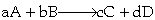The basis of calculation is always the limiting reactant. We will choose A as our basis of calculation and divide through by the stoichiometric coefficient to put everything on the basis of "per mole of A".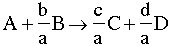The conversion X of species A in a reaction is equal to the number of moles of A reacted per mole of A fed, ie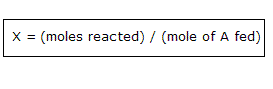Batch Flow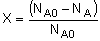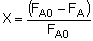What is the maximum value of conversion?

For irreversible reactions, the maximum value of conversion, X, is that for complete conversion, i.e. X=1.0.

For reversible reactions, the maximum value of conversion, X, is the equilibrium conversion, i.e. X=Xe.

Batch: Moles A remaining = NA = Moles A initially - Moles A reacted
NA= NA0 - moles A initially x (moles A reacted)/(moles A fed)

 NA = NA0 - NA0X

Flow: Rate of Moles of A leaving FA = Rate of Moles of A fed - Rate of Moles of A reacted =

FA= FA0 - Rate of moles A fed x (moles A reacted)/(moles A fed)
 FA = FA0 - FA0X

 Design Equations (p. 34-41) top

The design equations presented in Chapter 1 can also be written in terms of conversion. The following design equations are for single reactions only. Design equations for multiple reactions will be discussed later.

#### Reactor Mole Balances in Terms of Conversion (Click on Reactor to see picture)

Reactor Differential Algebraic Integral
Batch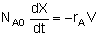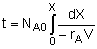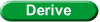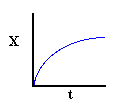CSTR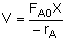PFR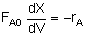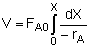PBR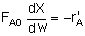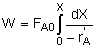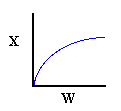Reactor Sizing (p. 41-56) top

By sizing a chemical reactor we mean we're either detering the reactor volume to achieve a given conversion or determine the conversion that can be achieved in a given reactor type and size. Here we will assume that we will be given -rA= f(X) and FA0. In chapter 3 we show how to find -rA= f(X).

Given -rA as a function of conversion,-rA=f(X), one can size any type of reactor. We do this by constructing a Levenspiel plot. Here we plot either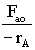or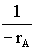as a function of X. Forvs. X, the volume of a CSTR and the volume of a PFR can be represented as the shaded areas in the Levenspiel Plots shown below: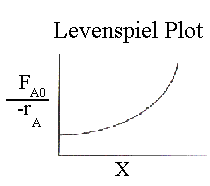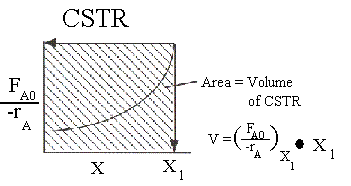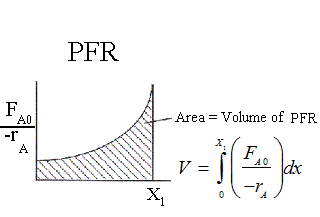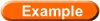Using the Ideal Gas Law to Calculate CA0 and FA0Levenspiel plots in terms of concentrations

 Numerical Evaluation of Integrals (Appendix A.4) top

The integral to calculate the PFR volume can be evaluated using a method such as Simpson's One-Third Rule (pg 1014):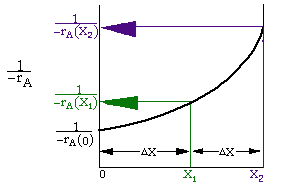NOTE: The intervals (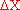) shown in the sketch are not drawn to scale. They should be equal.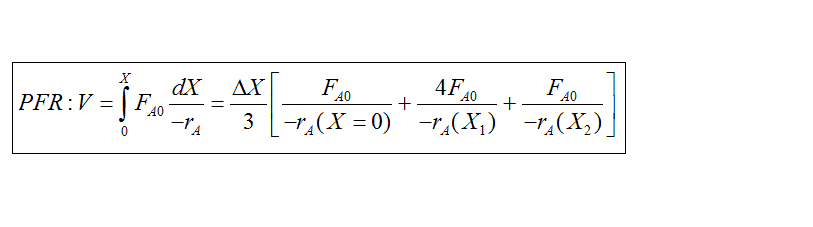Simpson's One-Third Rule is one of the more common numerical methods. It uses three data points. Other numerical methods (see Appendix A, pp 1009-1015) for evaluating integrals are:

1. Trapezoidal Rule (uses two data points)

2. Simpson's Three-Eighth's Rule (uses four data points)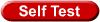Numerical Evaluation of an Integral

 Reactors in Series top

Given -rA as a function of conversion, one can also design any sequence of reactors: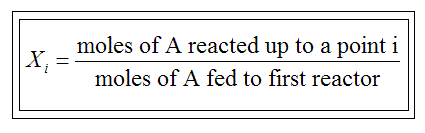Only valid if there are no side streams

Consider a PFR between two CSTRs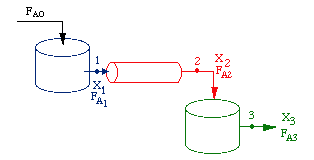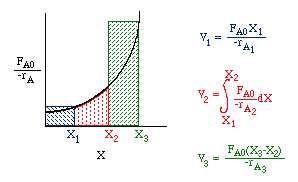Use Levenspiel plots to calculate conversion from known reactor volumes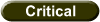One of the goals of the course is to have the readers further develop their critical thinking skills. One way to achieve this goal is through Socratic questioning. Throughout the course students will be asked to write questions on critical thinking drawing from information the Preface section B2. Below are some examples of critical thinking questions (CTQ) that are either superficial or don't use R. W. Paul's Six Types of Socratic QuestioningReactors in Series CSTR-PFR-CSTROld exam questions

 Space Time (p. 57) top

The Space time, tau, is obtained by dividing the reactor volume by the volumetric flow rate entering the reactor: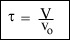Space time is the time necessary to process one volume of reactor fluid at the entrance conditions. This is the time it takes for the amount of fluid that takes up the entire volume of the reactor to either completely enter or completely exit the reactor.

Sample Industrial Space Times
 Reaction Reactor Temperature Pressure atm Space Time (1) C2H6 → C2H4 + H2 PFR 860°C 2 1 s (2) CH3CH2OH + HCH3COOH → CH3CH2COOCH3 + H2O CSTR 100°C 1 2 h (3) Catalytic cracking PBR 490°C 20 1 s < τ < 400 s (4) C6H5CH2CH3 → C6H5CH = CH2 + H2 PBR 600°C 1 0.2 s (5) CO + H2O → CO2 + H2 PBR 300°C 26 4.5 s (6) C6H6 + HNO3 → C6H5NO2 + H2O CSTR 50°C 1 20 minWhat's wrong with this solution?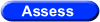Objective Assessment of Chapter 2